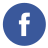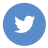### java primitive type

java

In Java, there are eight primitive data types:

1. boolean: a boolean value (true or false)
2. char: a single character
3. byte: a 8-bit signed integer
4. short: a 16-bit signed integer
5. int: a 32-bit signed integer
6. long: a 64-bit signed integer
7. float: a single-precision 32-bit floating-point number
8. double: a double-precision 64-bit floating-point number

These data types are called “primitive” because they are built-in to the Java language and are not objects. They are often used to store simple values such as numbers and characters.

For example, here is how you can declare and initialize variables of various primitive data types in Java:

``````boolean flag = true;
char ch = 'A';
byte b = 100;
short s = 10000;
int i = 1000000;
long l = 1000000000L;  // The "L" suffix indicates a long literal
float f = 3.14f;  // The "f" suffix indicates a float literal
double d = 3.14;``````